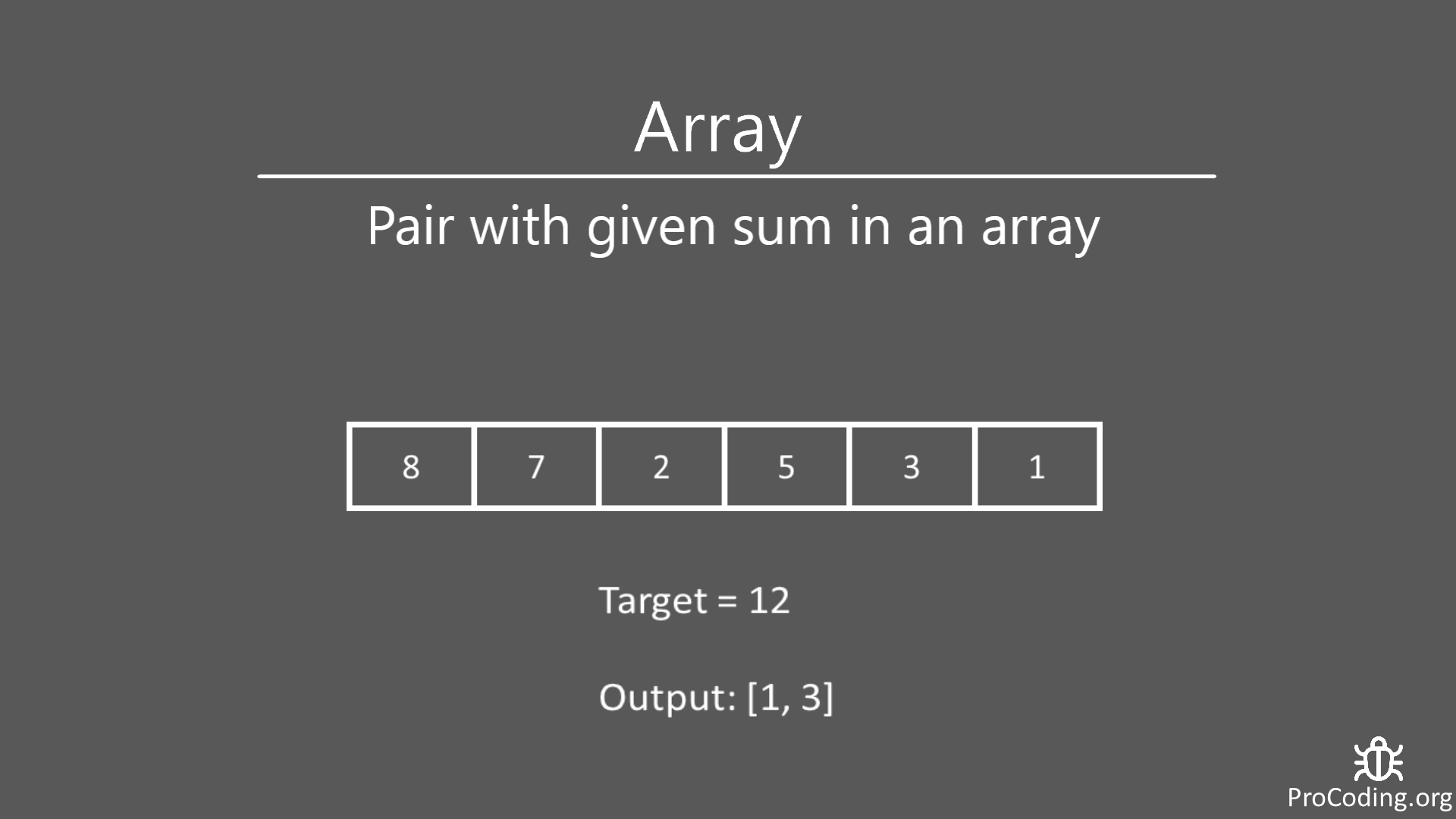# Pair with given sum in an array

Abhishek Kumar Category: Data StructuresTags: datastructures array

In this problem, we have to find a pair of number in an unsorted array which adds up to the given target value and return the index of both the numbers.### Problem

Find the pair of number in an unsorted array which adds up to the given target value and return index of both the numbers.

##### Example -
```Input: array=[8, 7, 2, 5, 3, 1]  target=12
Output: [1, 3]

Input: array=[1, 2, 3, 4, 5] target=9
Output: [3, 5]```

### Brute-force approach

We can solve this problem using nested loops but the time complexity will be O(n2), which is not an optimized solution.

``````def find_pair(nums, target):
for i in range(len(nums)):
for j in range(i+1, len(nums)):
if nums[i] + nums[j] == target:
return [i, j]
return None

nums = [8, 7, 2, 5, 3, 1]
target = 12

print(find_pair(nums, target))``````
``````function findPair(nums, target) {
for (let i = 0; i < nums.length; i++) {
for (j = i + 1; j <= nums.length; j++) {
if (nums[i] + nums[j] == target) {
return [i, j];
}
}
}
return null;
}

nums = [8, 7, 2, 5, 3, 1];
target = 12;

console.log(findPair(nums, target));``````

Output

`[1, 3]`

### Optimized solution (using HashMap)

We can use some data structure to store previous results which are already traversed and compare it to get the desired result. The data structure should be HashMap or any other which stores data in a similar way.

Fot this solution time complexity will be O(n).

``````def find_pair(nums, target):
hash_map = {}
for i in range(len(nums)):
if target - nums[i] in hash_map:
return [hash_map[target - nums[i]], i]
hash_map[nums[i]] = i
return None

nums = [8, 7, 2, 5, 3, 1]
target = 12

print(find_pair(nums, target))``````
``````function findPair(nums, target) {
const hashMap = {};
for (i = 0; i < nums.length; i++) {
if (target - nums[i] in hashMap) {
return [hashMap[target - nums[i]], i];
}
hashMap[nums[i]] = i;
}
return null;
}

nums = [8, 7, 2, 5, 3, 1];
target = 12;

console.log(findPair(nums, target));``````

#### Output

Output

`[1, 3]`

AUTHOR### Abhishek Kumar

Software engineer | Blogger | Keen learner
Python | Django | JavaScript | React | Next.js | Express.js | C
Passionate about learning and experimenting with new things and open to more opportunities and collaborations.